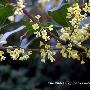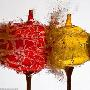# 桂花史話，桂花品種，桂花圖片欣賞「一年四季綠葉挂，八月枝頭開黃花。無風自有香味來，濃濃烈烈令人誇。」朋友，你知道這是什麽花嗎？這是桂花。740)then(this.width=740)" border=0740)then(this.width=740)" border=0740)then(this.width=740)" border=0740)then(this.width=740)" border=0740)then(this.width=740)" border=0740)then(this.width=740)" bord...查看完整版>>桂花圖片